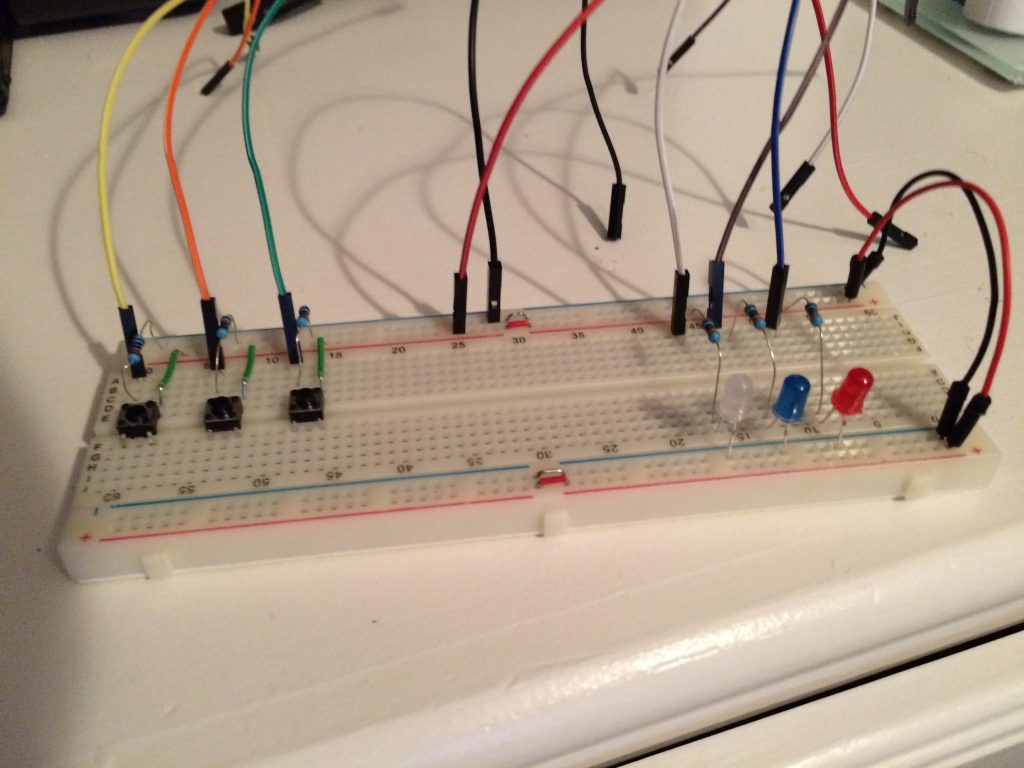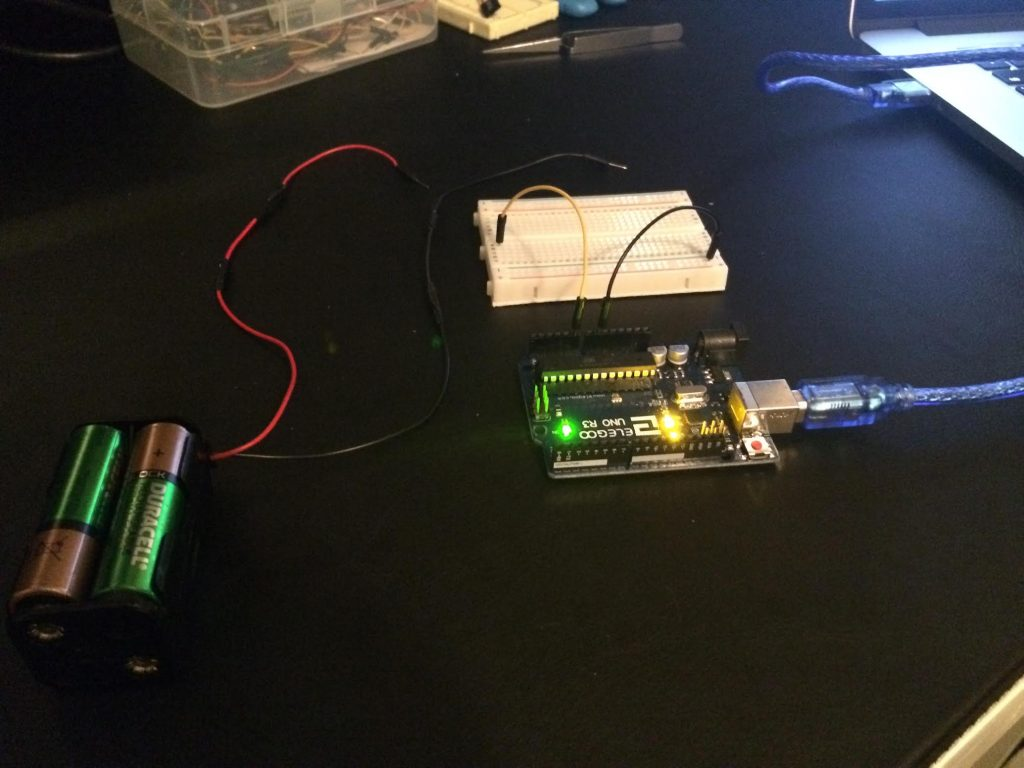## Python says, Simon’s hipster brother

Many of you may remember playing with a Simon Electronic Memory Gamewhen you were younger, you know something that looks like this:At it’s core the game is rather simple, the device lights up random colors, and you need to repeat the pattern. Of course it gets harder the longer you play.

I thought it would be fun to build a Simon game using Raspberry Pi and a few electronic components:

I used the following components to assemble the project:

• Raspberry Pi 3
• 3x 330 Ohm resistor
• 3x 1k Ohm resistor
• White LED
• Blue LED
• Red LED
• Assortment of wires

Here is a close up of the bread board and components:The Raspberry Pi’s GPIO pins are then connected to the bread board,
and a small Python script powers the Simon game:

```
from RPi import GPIO

from sys import exit
from random import choice
from time import sleep

# define our pins for leds
white = 14
blue = 15
red = 18

# define our pins for buttonss
white_button = 21
blue_button = 20
red_button = 16

# disable warnings
GPIO.setwarnings(False)

# set the board to use broadcom pin numbering
GPIO.setmode(GPIO.BCM)

# setup our LED pins as output
GPIO.setup(white, GPIO.OUT)
GPIO.setup(blue, GPIO.OUT)
GPIO.setup(red, GPIO.OUT)

# setup our buttons as input
GPIO.setup(white_button, GPIO.IN)
GPIO.setup(blue_button, GPIO.IN)
GPIO.setup(red_button, GPIO.IN)

# create empty pattern list for simon says game
pattern = []

# create a list of our choices for simon says game
choices = [white, blue, red]

# starting difficulty based on blink durations
duration = 0.75

"""
Append a random color to our pattern list
"""

color = choice(choices)
pattern.append(color)

def get_button():
"""
Gets the next button press and returns
"""

while True:
if GPIO.input(white_button):
return white

if GPIO.input(blue_button):
return blue

if GPIO.input(red_button):
return red

"""
"""

GPIO.output(led, GPIO.HIGH)
sleep(duration)
GPIO.output(led, GPIO.LOW)

"""
Blinks our pattern using duration as waits
"""

for led in pattern:
sleep(duration)

def check_pattern():
"""
Checks our button presses against pattern
"""

for led in pattern:
if led != get_button():
return False
sleep(0.3)  # delay so button press doesn't overlap
return True

def game_over():
"""
Game over function
"""

print 'Pattern Length: {}'.format(len(pattern))
print '''
_____          __  __ ______    ______      ________ _____
/ ____|   /\   |  \/  |  ____|  / __ \ \    / /  ____|  __ \
| |  __   /  \  | \  / | |__    | |  | \ \  / /| |__  | |__) |
| | |_ | / /\ \ | |\/| |  __|   | |  | |\ \/ / |  __| |  _  /
| |__| |/ ____ \| |  | | |____  | |__| | \  /  | |____| | \ \
\_____/_/    \_\_|  |_|______|  \____/   \/   |______|_|  \_\

'''

# blink all leds to show game over
for _ in range(3):
for c in choices:

exit()

if __name__ == '__main__':

# populate initial pattern

while True:

# check if our inputs were correct, else end game
if not check_pattern():
game_over()

# add a new color to pattern

# decrease our duration to increase difficulty
if duration > 0.05:
duration -= 0.07

```

Happy Hacking!

## Arduino values to Python over Serial

I’ve done a little bit of reading on the ReadAnalogVoltage of Arduino’s home page, and they give a straight forward way to read voltage from an analog pin.

I wanted to take this one step further and send the value over serial, then read it in Python using pySerial.

My setup is very straight forward, I have a Arduino UNO, a bread board, and a battery pack holding 4x AA batteries:To start out I want to merely print the voltage value in Arduino Studio to the serial console, my code looks something like this:

```void setup() {
// connect to serial
Serial.begin(9600);
}

void loop() {

// read value from analog pin

// convert to voltage and print to serial connection
float voltage = sensorValue * ( 5.0 / 1023.0 );
Serial.println(voltage);

}
```

Now that we’ve verified this works, lets make a couple modification to the Arduino code.

Since the value of the analogRead may be over 255 (more than can fit in a single byte), we will need to send two bytes, a high byte, and a low byte. This concept is called most significant byte, and least significant byte.

```void setup() {
// connect to serial
Serial.begin(9600);
}

void loop() {

// read value from analog pin

// get the high and low byte from value
byte high = highByte(sensorValue);
byte low = lowByte(sensorValue);

// write the high and low byte to serial
Serial.write(high);
Serial.write(low);

}
```

Then on the Python side we can use pySerial to read two bytes, and convert using the formula Arduino gave us.

```import serial

# open our serial port at 9600 baud
dev = '/dev/cu.usbmodem1411'
with serial.Serial(dev, 9600, timeout=1) as ser:

while True:

# read 2 bytes from our serial connection

if raw:

# read the high and low byte
high, low = raw

# add up our bits from high and low byte
# to get the final value
val = ord(high) * 256 + ord(low)

print round(val * ( 5.0 / 1023.0), 2)
```

One thing to take into consideration is, if we do not have voltage sent to the analog pin the result will be random and invalid. You will see this in the video before I connect the battery pack. Keep in mind my battery pack is producing about 5 volts:

## Python and sentiment analysis

While looking for datasets to throw at sklearn, I came across UCI Sentiment Labelled Sentences Data Set.

UCI is providing us with positive / negative tagging on real world data, the data comes from three sources (Amazon, Yelp, and IMDB).

The only problem is the format is a little strange.. We have a .txt file for each source, this is a raw unstructured  formatting, plus not every line is tagged with sentiment.

To make the data easier to interact with, I generated a json file with only the results containing sentiment. Go ahead and download it.

```\$ zcat sentiment.json.gz | head -n 25
[
{
"result": 0,
"source": "amazon_cells_labelled.txt",
"label": "negative",
"text": "So there is no way for me to plug it in here in the US unless I go by a converter."
},
{
"result": 1,
"source": "amazon_cells_labelled.txt",
"label": "positive",
"text": "Good case, Excellent value."
},
{
"result": 1,
"source": "amazon_cells_labelled.txt",
"label": "positive",
"text": "Great for the jawbone."
},
{
"result": 0,
"source": "amazon_cells_labelled.txt",
"label": "negative",
"text": "Tied to charger for conversations lasting more than 45 minutes.MAJOR PROBLEMS!!"
},```

Lets jump into an IPython interrupter and load the data:

```In : import json

Now that we have the data as a Python dictionary, create a DataFrame in the proper format:

```In : data = get_data_frame('sentiment.json')

In : data.shape
Out: (3000, 2)```

Next lets split our full dataset into a training, and testing dataset:

```In : train, test = get_train_test_data(data, size=0.2)

In : train.shape
Out: (2400, 2)

In : test.shape
Out: (600, 2)```

We are now set to run a bit of accuracy testing:

```In : test_predict(train, test)
Out: {
'test_score': 0.80000000000000004,
'train_score': 0.98375000000000001
}```

We can slice our full dataset a few more times, just to make sure our accuracy test is.. accurate:

```In : train, test = get_train_test_data(data, size=0.2)

In : test_predict(train, test)
Out: {
'test_score': 0.79000000000000004,
'train_score': 0.98416666666666663
}

In : train, test = get_train_test_data(data, size=0.2)

In : test_predict(train, test)
Out: {
'test_score': 0.80666666666666664,
'train_score': 0.98291666666666666
}

In : train, test = get_train_test_data(data, size=0.5)

In : test_predict(train, test)
Out: {
'test_score': 0.79466666666666663,
'train_score': 0.98999999999999999
}```

All that is left is to feed in the entire dataset and predict on new sentences:

```In : predict(data, 'This was the worst experience.')
Out: [
(u'positive', 0.17704535094140364),
(u'negative', 0.82295464905859583)
]

In : predict(data, 'The staff here was fabulous')
Out: [
(u'negative', 0.20651083543376234),
(u'positive', 0.79348916456623764)
]```
```In : predict(data, 'I hate you')
Out: [
(u'positive', 0.22509671479185445),
(u'negative', 0.77490328520814555)
]

In : predict(data, 'I love you')
Out: [
(u'negative', 0.10593166714256422),
(u'positive', 0.89406833285743614)
]```

Lets put it all together by looking at the Python functions:

```
import re
import json
import random
import string

from pandas import DataFrame, concat

from sklearn.pipeline import Pipeline
from sklearn.naive_bayes import MultinomialNB
from sklearn.neighbors import NearestNeighbors
from sklearn.cross_validation import train_test_split
from sklearn.feature_extraction.text import TfidfTransformer, \
CountVectorizer

# update the pipeline to get best test results!
steps = [
('count_vectorizer',  CountVectorizer(
stop_words='english', ngram_range=(1,  2))),
('tfidf_transformer', TfidfTransformer()),
('classifier',        MultinomialNB())
]

def id_generator(size=6):
"""
Return random string.
"""
chars = string.ascii_uppercase + string.digits
return ''.join(random.choice(chars) for _ in range(size))

def get_data_frame(filename):
"""
Read tweets.json from directory and return DataFrame.
"""

raw = dict(ids=[], text=[])

# open file and read as json
_data = open(filename)

# loop over all tweets in json file
for d in data:

# update raw list with tweet values.
raw['text'].append(
dict(text=d['text'], classification=d['label']))
raw['ids'].append(
id_generator(size=12))

return DataFrame(raw['text'], index=raw['ids'])

def merge(*args):
"""
Merge two or more DataFrames.
"""
return concat(args)

def get_train_test_data(data, size=0.2):
"""
Split DataFrame and return a training and testing set.
"""
train, test = train_test_split(data, test_size=size)
return train, test

def test_predict(train, test):
"""
Run predictions on training and test data,
then return scores.
"""

pipeline = Pipeline(steps)
pipeline.fit(train.text.values, train.classification.values)

train_score = pipeline.score(
train.text.values, train.classification.values)
test_score = pipeline.score(
test.text.values, test.classification.values)

return dict(train_score=train_score, test_score=test_score)

def predict(data, text):

pipeline = Pipeline(steps)
pipeline.fit(data.text.values, data.classification.values)

res = zip(pipeline.classes_, pipeline.predict_proba())
return sorted(res, key=lambda x:x)

```